Pandas Dataframe Examples: Manipulating Date and Time

# Pandas Dataframe Examples: Manipulating Date and Time

Last updated:

For information on the advanced Indexes available on pandas, see Pandas Time Series Examples: DatetimeIndex, PeriodIndex and TimedeltaIndex

Full code available on this notebook

## String column to datetime

Use pd.to_datetime(string_column):

import pandas as pd

df = pd.DataFrame({
'name': ['alice','bob','charlie'],
'date_of_birth': ['10/25/2005','10/29/2002','01/01/2001']
})

df['date_of_birth'] = pd.to_datetime(df['date_of_birth'])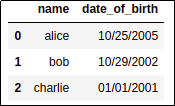BEFORE: column is of type 'object'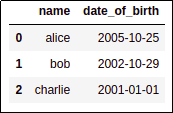AFTER: column 'date_of_birth' is
now of type 'datetime' and you can
perform date arithmetic on it

## String column to datetime, custom format

For custom formats, use format parameter:

See all formats here: python strftime formats

import pandas as pd

df = pd.DataFrame({
'name': ['alice','bob','charlie'],
'date_of_birth': ['27/05/2001','16/02/1999','25/09/1998']
})

df['date_of_birth'] = pd.to_datetime(df['date_of_birth'],format='%d/%m/%Y')BEFORE: In some countries, dates are often
displayed in a day/month/year
format
(date_of_birth is of type string)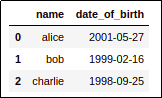AFTER: Just pass the format parameter so that
pandas knows what format your
dates are in
(date_of_birth is now of type datetime)

## Pandas timestamp now

Use pd.Timestamp(datetime.now()):

from datetime import datetime
import pandas as pd

# some dataframe
df = pd.DataFrame(...)

df["datetime"] = pd.Timestamp(datetime.now())


## Pandas timestamp to string

See available formats for strftime here

Use .strftime(<format_str>) as you would with a normal datetime:

EXAMPLE: format a Timestamp column in the format "dd-mm-yyyy"

import pandas as pd

df = pd.DataFrame({
"name":["alice","bob","charlie", "david"],
"age":[12,43,22,34]
})

# a timestamp column
df["timestamp_col"] = pd.Timestamp(datetime.now())

# use strftime to turn a timestamp into a
# a nicely formatted d-m-Y string:
df["formatted_col"] = df["timestamp_col"].map(lambda ts: ts.strftime("%d-%m-%Y"))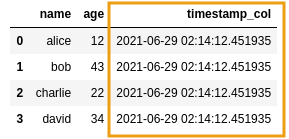BEFORE: a dataframe with a timestamp column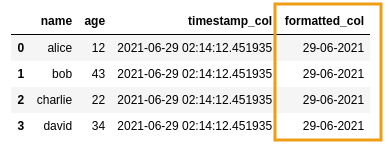AFTER: added a new string column with a
formatted date

## Filter rows by date

Only works for columns of type datetime (see above)

For example: Filter rows where date_of_birth is smaller than a given date.

Use pandas.Timestamp(<date_obj>) to create a Timestamp object and just use < operator:

import pandas as pd

from datetime import date

df = pd.DataFrame({
'name': ['alice','bob','charlie'],
'date_of_birth': ['10/25/2005','10/29/2002','01/01/2001']
})

# convert to type datetime
df['date_of_birth'] = pd.to_datetime(df['date_of_birth'])

df[df['date_of_birth'] < pd.Timestamp(date(2002,1,1))]

dfBEFORE: original dataframe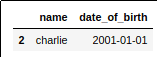AFTER: only charlie was born prior to 1/1/2002

## Filter rows where date in range

import pandas as pd
from datetime import date

date_from = pd.Timestamp(date(2003,1,1))
date_to = pd.Timestamp(date(2006,1,1))

# df is defined in the previous example

df = df[
(df['date_of_birth'] > date_from ) &
(df['date_of_birth'] < date_to)
]

dfBEFORE: original dataframe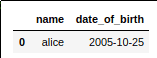AFTER: only alice's date of birth is
between 2003/01/01 and 2006/01/01

## Group by year

Naturally, this can be used for grouping by month (), day of week, etc

Create a column called 'year_of_birth' using function strftime and group by that column:

import pandas as pd

df = pd.DataFrame({
'name': ['alice','bob','charlie', 'david'],
'date_of_birth': ['2001-05-27','1999-02-16','1998-09-25', '1999-01-01']
})

df['date_of_birth'] = pd.to_datetime(df['date_of_birth'])

# step 1: create a 'year' column
df['year_of_birth'] = df['date_of_birth'].map(lambda dt: dt.strftime('%Y'))

# step 2: group by the created column
df.groupby('year_of_birth').size()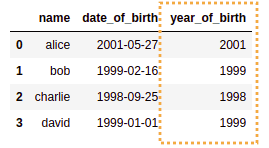STEP 1: Add a new column to
the original dataframe,
called year_of_birth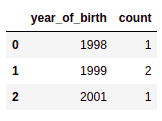STEP 2: grouping b year_of_birth,
you get the number of rows per year

## Group by start of week

If you just change group-by-year to week, you'll end up with the week number, which isn't very easy to interpret.

Use dt - timedelta(dt.weekday()) to get the start of the week (Monday-based) and then group by:

from datetime import timedelta, date

import pandas as pd

df = pd.DataFrame({
'item':          ['a',          'b',         'c',         'd',          'e',          'f'],
'purchase_date': ['2001-01-15', '2001-01-18','2001-01-21','2001-01-24', '2001-01-27', '2001-01-30']
})

# convert values to datetime type
df['purchase_date'] = pd.to_datetime(df['purchase_date'])

# to have Sunday as the start of the week instead,
# use (dt - timedelta(days=dt.weekday() +1))
df['purchase_start_of_week'] = df['purchase_date'].map(lambda dt: dt - timedelta(days=dt.weekday()))

df.groupby('purchase_start_of_week').size()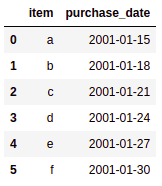BEFORE: Original dataframe with purchase_date
datetime column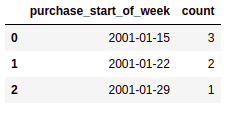AFTER: Count of purchases per week